# Plus Two Chemistry Chapter Wise Questions and Answers Chapter 3 Electrochemistry

Students can Download Chapter 3 Electrochemistry Questions and Answers, Plus Two Chemistry Chapter Wise Questions and Answers helps you to revise the complete Kerala State Syllabus and score more marks in your examinations.

## Kerala Plus Two Chemistry Chapter Wise Questions and Answers Chapter 3 Electrochemistry

### Plus Two Chemistry Electrochemistry One Mark Questions and Answers

Question 1.
The metal which acts as the anode in Daniell cell is _______
Zinc

Question 2.
Electrolysis of dilute H2SO4 liberates ________ gas at cathode.
Hydrogen

Question 3.
The charge required to reduce 1 mole of I2 to I is
(a) 96500 C
(b) 2 × 96500 C
(c) $$\frac{1}{2}$$ × 96500 C
(d) 4 × 96500 C
(b) 2 × 96500 CQuestion 4.
Of the following metals that cannot be obtained by electrolysis of the aqueous solution of their salts are
(a) Ag and Mg
(b) Ag and Al
(c) Mg and Al
(d) Cu and Cr
(c) Mg and Al

Question 5.
Chemically, rust is _______
Hydrated ferric oxide (Fe2O3.xH2O)

Question 6.
One of your friends argues that in the laboratory CuS04 is not stored in Aluminium bottles.

1. Do you agree?
2. Justify.

1. Yes.

2. Aluminium displaces copper from copper sulphate solution. Because, in electrochemical series Al stands before copper. The reduction potential of Cu is greater than that of Al. Greater the value of reduction potential, more easily is the substance reduced.
(Al+3/Al) = – 1.66 V,
(Cu+2/Cu)= + 0.34V

Question 7.
Arrange the following metals in the order in which they displace each other from the solution of their salts:
Al, Cu, Fe, Mg and Zn
The correct order is Mg, Al, Zn, Fe, Cu

Question 8.
The conductivity of 0.20 M solution of KCl at 298 K is 0.0248 S cm-1. Calculate the molar conductivity.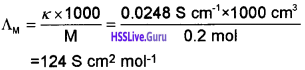Question 9.
The limiting molar conductivity of HCl, CH3COONa and NaCl are respectively 425, 90 and 125 mho cm2mol-1 at 25°C. The molar conductivity of 0.01 m. CH3COOH solution is 7.5 mho cm2 mol-1 at the same temperature. The degree of dissociation of 0.1 as acetic acid solution at the same temperature is _________
0.02

Question 10.
The ion of least limiting molar conductivity among the following is
(a) SO2-4
(b) H+
(c) Ca2+
(d) CH3COO
(d) CH3COOQuestion 11.
Standard electrode potential of three metals XY and Z are 0.52V, 2.87 V and -0.44 V respectively. The reducing power of these metals are
Y > Z > X

Question 12.
A current of strength of 9.65 ampere is passed through excess fused AlCl3 for 5 hours. How many liters of chlorine will be liberated at STP.
20.16

Question 13.
Consider the following four electrodes
P = Cu2+(.0001 M/Cu(s) Q = Cu2+(0.1 M)/Cu(s)
R = Cu2+(0.01 M)/Cu(s) S = Cu2+(0.001 M)Cu(s)
If the standard reduction potential of Cu2+/Cu is +.34V the reduction potential in volts of the above electrode follows the order.
Q > R > P

### Plus Two Chemistry Electrochemistry Two Mark Questions and Answers

Question 1.
Resistance of lead is greater than that of gold.

1. Do you agree?
2. What do you mean by resistance?
3. What do you mean by resistivity?

1. Yes.
2. Resistance is the hindrance offered by a substance to the flow of electricity through it.
3. Resistivity is the resistance of a conductor of length 1 cm and area of cross section 1 cm2.

R ∝ $$\frac{\ell}{\mathrm{A}}$$ Or R = ρ$$\frac{\ell}{\mathrm{A}}$$ where R → Resistance
and ρ → resistivity.
When l = 1 cm and A = 1 cm2
then, R = ?

Question 2.
In the chemistry lab, a student immersed two platinum electrodes in a solution of CuSO4 and electric current was passed through the solution. After sometime he noticed that the colour of CuSO4 disappeares with the evolution of a gas at the electrode. In his Practical Log, he recorded that the colourless solution contains H2SO4.

1. Is it true?

1. Yes.

2. When aqueous CuSO4 solution is electrolysed using Pt electrodes, Cu is deposited at the cathode and O2 is liberated at the anode.
CuSO4(aq) → Cu2+(aq) + SO42-(aq)
Cu2+(aq) + 2$$\bar { e }$$ → Cu(s) (at cathode)
H2O(l) → 2H+(aq) + 1/2 O2(g) + 2$$\bar { e }$$ (at anode)
Thus, H2SO4 is formed as the secondary product.

Question 3.
Consider the argument, “On the basis of position in the electrochemical series, Al does not displace hydrogen from water and acids

1. Do you agree with the argument?

1. No.
2. Metals which are above hydrogen in the electro chemical series can displace H2 from acids, i.e., elements having lower reduction potential than hydrogen, can liberate H2 from acids and water. Al displaces hydrogen from water and acids.Question 4.
How much electricity in Faraday is required to produce

1. 20.0 g of Ca from molten CaCl2?
2. 45.0 g of Al from molten Al2O3?

(Hint: Atomic masses – Ca: 40 u, Al: 27 u)
1. The electrode reaction is Ca2+(aq) + 2e → Ca(s)
Electricity required to produce 40 g Ca = 2 F
Electricity required to produce 20 g Ca = 1 F

2. The electrode reaction is.
Al3+(aq) + 3e → Al(s)
Electricity required to produce 27 g of Al = 3 F
Electricity required to produce 45 g Al = 5 F

Question 5.
In a debate, a student said “Electrolytes dissolved in water dissociate into constituent ions”. His friend supported this statement and said “The degree of dissociation of an electrolyte increase with the increasing concentration of the electrolyte.”

1. Do you agree with these statements?
2. If you agree or not, give the reason.

1. The first statement is correct, but the second statement is wrong.
2. Degree of dissociation of an electrolyte increases with dilution and not by increasing the concentration.

Question 6.
The standard electrode potential of the elements A, B and C are 0.68, – 2.50 and – 0.50 volts respectively.

1. Arrange them in the order of their reducing power.

1. B < C < A
2. Greater the value of reduction potential, greater will be the reducing power.

Question 7.
In a standard hydrogen electrode, is it possible to replace the Platinum wire in the glass tube with Aluminium wire? Justify your answer.
No. Platinum is an inert electrode and moreover the coefficient of cubical expansion of platinum is equal to that of glass. But, aluminium being an active metal will interfere in the cell reaction.

Question 8.
Listen to the following statements.
“Zinc displaces silver from AgNO3 solution, because standard oxidation potential of zinc is greaterthan that of silver”.

1. Is it true?
2. What happens when we use copper instead of zinc?

1. Yes.
2. Copper also displaces Ag from AgNO3 solution.
3. In electrochemical series, Cu lies above Ag. The reduction potential of copper is +0.34 V. But in the case of silver, the reduction potential is +0.80 V.Question 9.
“Metal having -ve reduction potential when dipped in the solution of its own ion has a tendency to go into the solution”.

1. Analyse the above statement.
2. Compare the reduction potential of H+ ion with that of other metal ions.

1. The statement is correct.
2. The reduction electrode potentials of elements lying above hydrogen in the electrochemical series are negative while those of elements lying below hydrogen are positive. The standard potential for the reaction 2H+ + 2e → H2(g) is taken as 0.00 V.

Question 10.
Consider the statement ‘Specific conductance increases on dilution’.

1. Is it true?
3. Define specific conductance and give its equation.

1. No.
2. This is because, the number of ions per unit volume that carry the current in a solution decreases on dilution.
3. Specific conductance/Conductivity of a conductor is the conductance offered by the conductor of unit length and unit area of cross section.

k = $$\frac{l}{R A}=\frac{1}{R} \times \frac{l}{A}$$ where $$\frac{l}{A}$$ is the cell constant.

Question 11.
Write Nernst equation and calculate the emf of the following cell at 298 K.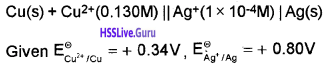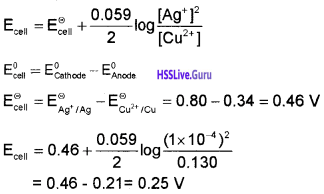Question 12.
The resistance of a conductivity cell containing 0.001 M KCl solution at 298 K is 1500 Ω. What is the cell constant if conductivity of 0.001 M KCl solution at 298 K is 0.146 × 10-3 S cm-1?
Cell constant = $$\frac{\text { Conductivity }}{\text { Conductance }}$$
i.e., Cell constant = Conductivity × Resistance
= 0.146 × 10-3 S cm-1 × 1500 Ω
= 0.219 cm-1

Question 13.

1. Give the name for the iron coated with the Zinc.
2. Can we store Cl2 gas in copper vessel? Give reason.
(E°(Cu2+/ Cu) = + 0.34 V E°(Cl2/Cl) = +1 -36 V)(2)
3. The equilibrium constant of a reaction which is difficult to calculate can be calculated by knowing the standard potential of the cell in which reaction takes place. Give the mathematical expression relating equilibrium constant and cell potential (2)

1. Galvanised iron
2. No. Since the reduction potential of chlorine is greater than that of copper, chlorine reacts with copper to form cuprous chloride. E°(Cl2/Cl) > (E°(Cu2+/ Cu))
3. cell = $$\frac{2.303 \mathrm{RT}}{\mathrm{nF}} \log \mathrm{K}_{\mathrm{c}}$$

where E°cell is the standard cell potential (E°cell = E°cathode – E°anode and Kc is the equilibrium constant of the cell reaction.

### Plus Two Chemistry Electrochemistry Three Mark Questions and Answers

Question 1.
Corrosion causes huge loss of metals.

1. Can you suggest two examples of corrosion from everyday life?
2. Suggest any two factors which enhance the rate of corrosion.
3. What is the chemical name of rust?

1. Rusting of iron, Tarnishing of silver
2. two factors which enhance the rate of corrosion.
• Presence of impurities
• Air and moisture
3. Hydrated ferric oxide (Fe2O3.xH2O)Question 2.
Write a note on primary cells, secondary cells and fuel cells.

 Primary cell Secondary cell Electrode reaction cannot be reversed. Electrode reaction can be reversed by an external electric energy source. Reaction occurs only once & after use they become dead; not chargeable. Reaction can occur many times in both directions; Rechargeable Can’t be reused Can be reused Cannot be recharged Can be recharged

Question 3.
Various factors influence the conductance of solutions.

1. What do you mean by conductance?
2. Write its formula.
3. Write the factors influencing it.

1. It is also defined as the reciprocal of resistance. It is denoted by C.

2. Conductance, C = $$\frac{1}{\mathrm{R}}$$
But, R = ρ$$\frac{l}{A}$$
∴ C = $$\frac{1}{\rho} \frac{\mathrm{A}}{\ell}$$

3. The factors influencing it.

• The nature of the electrolyte added.
• Nature of solvent and its viscosity.
• Concentration of the solution.
• Temperature.

Question 4.
The position of some metals in the electrochemical
series in the decreasing order of electropositive
character is given:
Mg > Al > Zn > Cu > Ag

1. What would happen if a copper spoon is used to stir a solution of aluminium?
2. Is there any reaction?
3. Comment on your response and justify.

1. Nothing will happen. The Al solution can be safely stirred using a Cu spoon.
2. No.
3. The Cu spoon will not react with the solution of Al. Greater the value of reduction potential of a metal, more easily it is reduced. The element having low reduction potential is more reactive and is oxidised. Cu has greater reduction potential than Al and is more active metal than Cu.

Question 5.
In the lab a student added a zinc piece to CuSO4 solution,

1. What may be his observation?
2. What did that observation indicate?
3. Give reason for the above observation.

[Hint: Process related to electrolysis]

1. Zinc pieces will dissolve in CuSO4 solution and the intensity of the blue colour of CuSO4 solution decreases.
2. Zinc displaces copper from copper sulphate solution.
3. Lower the value of reduction potential more easily is the substance oxidised and greater the value of reduction potential, more easily is the substance reduced. Zinc is more reactive. Hence Zinc displaces copper from copper sulphate solution.

Question 6.
Observe the given figure.

1. Identify the process.
2. Identify A and B.
3. Illustrate the mechanism of this process.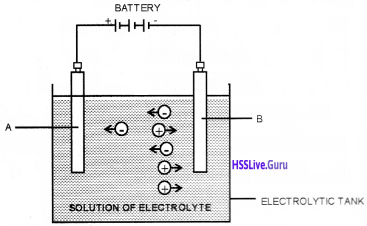1. Electrolysis.
2. A: Anode B: Cathode
3. During electrolysis, initially the electrolyte gets ionised. On passing electricity, the ions migrate to oppositely charged electrodes. Cations move towards cathode and anions move towards anode. Cations gain electrons from cathode while anions give out electrons to anode during discharge. The movement of ions in solution is responsible for conduction of electricity.Question 7.
In the Chemistry lab the teacher told the students to label some electrolytes suitably. The labelling given by one student is as given:
Strong electrolyte – H2SO4, NH4OH, KOH, Ca(OH)2
Weak electrolytes – NaOH, HCN, NaCl, HCl

1. Is it correct? Justify.
2. Correct it.
3. What do you mean by electrolysis?

1. No.
2. Strong electrolytes – H2SO4, KOH, HCl, NaOH, NaCl
Weak electrolytes – NH4OH, HCN, Ca(OH)2
3. The process of chemical decomposition of electrolyte by the passage of electricity through its molten or dissolved state is called electrolysis.

Question 8.
This figure shows the corrosion of iron.1. What happens to a metal during corrosion – oxidation or reduction?
2. Explain the electrochemical theory of rusting of iron.
3. Write two examples for metals which undergo corrosion.

1. Oxidation.

2. The impure surface of iron behaves like as electrochemical cell. Pure iron functions as the anode while the impure surface acts as cathode. The electrolyte is moisture containing dissolved O2 or CO2.
At anode: 2Fe(s) → 2Fe2+(aq) + 4e
At cathode: 4H+(eq) + O2(g) + 4e → 2H2O(l)
The overall reaction is
2Fe(s) + O2(g) + 4H+(aq) → 2Fe2+(aq) + 2H2O(l)
Ferrous ions so formed are oxidised by atmospheric oxygen to ferric ions. The rust formed is chemically hydrated ferric oxide (Fe2O3.xH2O)

2Fe2+(aq) + 2H2O + 1/2 O2 → Fe2O3 + 4H+
Fe2O3 + xH2O → Fe2O3.xH2O (rust)

3. Iron and Copper.

Question 9.
In an experimental set-up for the measurement of potential of a half-cell using the reference electrode and a salt bridge, the salt bridge was suddenly removed.

1. What happens to the voltage when the salt bridge is removed?
3. Write any two examples of inert electrolytes used for the construction of salt bridge.

1. Voltage ceases.
2. When the salt bridge is removed the migration of ions between the half cells is hindered which results in the accumulation of charge near the electrodes. This decreases the cell voltage.
3. KCl, NH4NO3Question 10.
In the class, teacher wrote some ions on the black board. It is given below.1. Which among the ions, gain electron more easily?
2. Is it true that the reduction potential of hydrogen is less than that of Na and Mg?

1. Mg2+
2. No. The reduction potential of hydrogen is greater than that of both Na and Mg.
3. In electrochemical series, sodium and magnesium lie above hydrogen. Standard reduction potential of hydrogen electrode is taken as zero volt.

Question 11.1. What does this figure represent?
2. What is its potential?
3. How can we construct this?

1. SHE (Standard Hydrogen Electrode) Or NHE (Normal Hydrogen Electrode).
2. Its potential is taken as zero.
3. It consists of a platinum wire sealed into a glass tube carrying a platinum foil, coated with finely divided Pt, at one end. The electrode is dipped in an aqueous acid solution of 1M concentration. Pure hydrogen gas at 1 bar pressure is bubbled through the solution at 298 K.

Question 12.
Given the standard electrode potential values
(Al3+/Al) = -1 -66 v E°(Zn2+/Zn) = -0.764 V.

1. Draw a neat diagram of galvanic cell using the above electrodes and write the cell reaction.
2. Calculate the emf of the above cell.

1.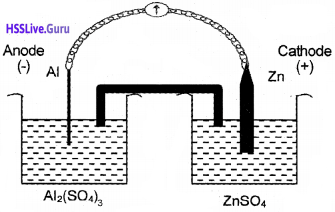2. Cell emf, Ecell = E°(Zn2+/Zn) – E°(Al3+/Al)
= – 0.764 – (-1.66) = + 0.896 V

Question 13.
Depict the galvanic cell in which the reaction Zn(s) + 2Ag+(aq) → Zn2+ + 2Ag(s) takes place. Further show:

1. Which of the electrodes is negatively charged?
2. The carriers of current in the cell.
3. Individual reaction at each electrode.

The electrochemical cell which will involve the given cell reaction is depicted as Zn(s) | Zn2+(aq) || Ag+(aq) | Ag(s)

1. In this cell, the electrode Zn(s) | Zn2+(aq) will act as negative terminal and Ag | Ag+(aq) electrode will act as positive terminal.
2. The conventional current will flow from silver to zinc electrode in the external circuit.
3. Individual reaction at each electrode.
• Cathodic reaction: 2Ag+(aq) + 2e → 2Ag(s)
• Anodic reaction: Zn(s) → Zn2+(aq) + 2e

Question 14.
How much electricity in terms of Faraday is required to produce

1. 20.0 g of Ca from molten CaCl2?
2. 40.0 g of Al from molten Al2O3?

1. The electrode reaction is
Ca2++ 2e → Ca
Electricity required to produce 40 g of Ca = 2 F
Electricity required to produce 20 g of Ca = 1 F

2. The electrode reaction is Al3+ + 3e → Al
Electricity required to produce 27 g of Al = 3 F
Electricity required to produce 40 g of Al = $$\frac{3 \mathrm{F} \times 40}{27}$$ = 4.44 FQuestion 15.
We can calculate the molar conductivity of NH4OH at infinite dilution if Λ° of Ba(OH)2, BaCl2 and NH4Cl are known.

1. Can you agree with this statement? Explain the law behind it.
2. Give the equation for Λ° of NH4OH from the above data.

1. Yes. According to Kohlrausch’s law the molar conductivity of an electrolyte at infinite dilution is equal to the sum of the molar ionic conductivities of the cations and anions at infinite dilution.

2.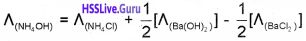Question 16.

1. Represent the cell in which the following reaction takes place.
Mg(s) + 2Ag2+(0.0001M) → Mg2+(0.130M) + 2 Ag(s). Calculate its Ecell if E°cell = + 3.17 V.
2. Discuss the variation of molar conductivity with concentration for acetic acid and KCI.

1. Mg(s) | Mg2+(0.130M) || Ag+(0.0001M) | Ag(s)

2. Conductivity decreases with dilution because the number of ions per unit volume that carry the current in a solution decreases on dilution. For strong electrolytes molar conductivity increases steadily with increase in dilution due to decrease in interionic attraction. For weak electrolytes molar conductivity increases with dilution due to increase in degree of dissociation.Question 17.

1. Write the relation between molar conductance and molarity of an electrolyte solution.
2. λm of NaCl, HCl and CH3COONa are 126.4, 425.9 and 91 S cm2 mol-1 respectively. Calculate the λm of acetic acid.

1. Λm = $$\frac{1000 \mathrm{k}}{\mathrm{M}}$$

2. Λ°CH3COOH = Λ°CH3COONa + Λ°HCl – Λ°NaCl
= 91 + 425.9 – 126.4
= 390.5 Ω-1 cm2 mol-1

Question 18.
Certain cells are given below.

1. Classify them into primary, secondary and fuel cells. (Mercury cell, Nickel-Cadmium cell, Lead storage battery, H2- O2 cell, Dry cell)
2. Represent the cell in which the overall reaction is,
Cd + 2Ag+ → Cd2+ + 2Ag

1. Primary cells: Mercury cell, Dry cell
Fuel cell : H2 – O2 cell

2. Cd → Cd2+ + 2e (Oxidation half cell)
2Ag+ + 2e → 2Ag (Reduction half cell)
Therefore the cell is represented as Cd | Cd2+ || Ag+ | Ag

### Plus Two Chemistry Electrochemistry Four Mark Questions and Answers

Question 1.
Every conductivity cell has a definite value for its cell constant.

1. What is cell constant?
2. How is it related with specific conductance?
3. Calculate the cell constant of a conductivity cell containing 0.05M KCl solution, whose resistance is 100 ohm and conductivity is 0.0075 ohm-1 cm-1.

1. The quantity $$\ell / \mathrm{A}$$ is called cell constant.
A = Area of cross section of the electrodes.
$$\ell$$ = distance of separation of two electrodes.

2. Specific conductance = Cell constant × Conductance

3. Cell constant = Specific conductance × Resistance
= 0.0075 ohm 1 cm-1 × 100 ohm = 0.75 cm-1Question 2.

1. Explain the working of a H2 – O2 fuel cell.
2. What are the advantages of fuel cells over other cells?

1. In H2 – O2 fuel cell, hydrogen and oxygen are bubbled through porous carbon electrodes into concentrated aqueous NaOH solution, which acts as the electrolyte. The electrode reactions are,
Anode: 2H2(g) + 4 OH(aq) → 4 H2O(l) + 4 $$\bar { e }$$
Cathode: O2(g) + 2 H2O(l) + 4 $$\bar { e }$$ → 4 OH(aq)
Overall reaction: 2H2(g) + O2(g) → 2 H2O(l)

2. The advantages of fuel cells over other cells

• They are pollution free.
• More efficient than conventional methods.
• Runs continuously as long as the reactants are supplied.
• Electrodes are not affected.

Question 3.
Consider the equation, λmHCl = λmH+ + λmCl

1. Is the above equation correct?
2. Explain the law.
3. Write the application of this law.

1. The above equation is correct.

2. Kohlrausch’s law. Molar conductance at infinite dilution of an electrolyte is the sum of the molar ionic conductivities at infinite dilution of the cations and anions present in the solution.

3. The application of this law:

• To find the molar conductivity at infinite dilution (Λ°m) of weak electrolytes.
• To find the degree of dissociation (α) of weak electrolytes.
α = $$\frac{\Lambda_{m}}{\Lambda_{m}^{0}}$$
• To determine the dissociation constant of weak electrolytes.
Ka = $$\frac{\mathrm{c}_{\alpha}^{2}}{1-\alpha}$$

Question 4.
1. Depict the galvanic cell in which the reaction Zn(s) + 2Ag+(aq) → Zn2+(aq) + 2Ag(s) takes place.
2. Further show

• Which of the electrodes is negatively charged?
• The carriers of current in the cell.
• Individual reaction at each electrode.

1. Zn(s) | Zn2+(aq) || Ag+(aq) | Ag(s)

2. Further show

• Zn electrode is negatively charged (Anode).
• Ions
• Individual reaction at each electrode.
• At anode : Zn(s) → Zn2+(aq) + 2e
• At cathode : 2Ag+(aq) + 2e → 2Ag(s)Question 5.

1. It is not possible to determine the molar conductivity of weak electrolytes at infinite dilution graphically. Do you agree with this? Justify your answer.
2. Calculate the molar conductivity of NH4OH at infinite dilution if molar conductivity at infinite dilution of Ba(OH)2, BaCl2, and NH4Cl are 523.4, 280 and 130 ohm-1 cm2 mol-1 respectively.

1. Yes. I agree with this statement because for weak electrolytes such as acetic acid, molar conductivity increases rapidly with dilution.
Hence, the plot of Λm against $$\sqrt{\mathrm{C}}$$ (C = concentration of electrolyte) is not linear. Therefore the value of Λ°m for weak electrolytes cannot be obtained by extraploting the graph.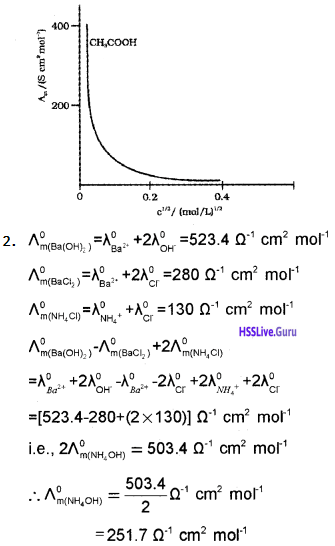Question 6.
Standard reduction potentials of some elements are given below.
Al = – 1.66 V, Cd = – 0.40 V, Ag = + 0.80 V & Au = + 1.36 V

1. Which of these can displace hydrogen from hydrochloric acid. Why?
2. Construct a galvanic cell using any two electrodes. Write the cell reaction and calculate the standard emf.

1. Al and Cd can displace hydrogen from HCl. Metals with low reduction potential than H (i.e., with -ve value) can liberate H2 from acids.

2.Question 7.
Molar conductance at infinite dilution of acetic acid cannot be determined experimentally.

1. How can it be determined?
2. State the law.
3. Give two sets of electrolytes which can be used to find out the molar conductance of acetic acid at infinite dilution.

1. It can be determined using Kohlrausch’s law of independent migration of ions.
2. Molar conductivity at infinite dilution of an electrolyte is the sum of molar ionic conductivities of the cation and anion at infinite dilution.
3. CH3COONa, HCl, NaCl & CH3COOK, HCl, KCl.

Question 8.
An electrochemical cell is a combination of two half cells consisting of a metal with its own ions in solution.

1. Represent the Daniell cell.
2. cathode and E°anode for the above cell are + 0.34 V and – 0.76 V respectively. Calculate the E°cell?
3. Give the graphical interpretation of the following graph.1. Zn(s) | Zn2+(aq) || Cu2+(aq) | Cu(s)
2. cell = E°cathode – E°anode = (-0.34V) – (-0.76V) = +1.1V
3. The graph indicates the variation of conductance with dilution for strong and weak electrolytes. For both of them Λm increases with dilution. For strong electrolytes like KCl, the graph is a straight line since the variation is linear. But for weak electrolytes like CH3COOH the graph is a curve due to step increase in the value of molar conductance near infinite dilution.

Question 9.
The standard electrode potential of some electrodes are given below.

• Ag+/Ag = + 0.80 V
• Fe2+/Fe = – 0.44 V
• Cu2+/Cu = + 0.34 V
• Al3+/Al = – 1.66 V

Construct 4 possible electrochemical cells and calculate the cell potential of the corresponding cells?Question 10.

1. What do you mean by cells?
2. What is the difference between primary cells and secondary cells?
3. Fill in the following table: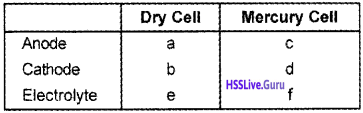1. Cells are the devices in which chemical energy is converted into electrical energy.

2.

 Primary cell Secondary cell Electrode reaction cannot be reversed. Electrode reaction can be reversed by an external electric energy source. Reaction occurs only once & after use they become dead; not chargeable. Reaction can occur many times in both directions; Rechargeable Can’t be reused Can be reused Cannot be recharged Can be recharged

3.

• a – Zinc
• b – Graphite
• c – Zinc-Amalgam
• d – HgO and Carbon
• e – Paste of NH4Cl and ZnCl2
• f – Paste of KOH and ZnOQuestion 11.
Iron gets destroyed due to continuous contact with the environment.

1. Name the phenomenon.
2. Suggest some prevention methods.
3. Explain any one prevention method

1. Corrosion

2. Some prevention methods

• By using non-metallic coating
• By using metallic coating
• Cathodic protection
• Alloy formation

3. Metallic coating or electroplating iron is coated with zinc. Iron thus obtained is called galvanised iron. Coating of an electropositive metal on the metal surface.

Question 12.
Corrosion causes enormous damage to various articles made of metal.

1. What do you mean by corrosion?
2. What is barrier protection?
3. Name any two metals which can be used as sacrificial electrodes in the electrochemical method of protecting iron.

1. Destruction of a metal.

2. In this method, a barrier film is introduced between metal and atmospheric oxygen and moisture. Painting or with a thin film of oil or grease electroplaing.

3. Two metals which can be used as sacrificial electrodes in the electrochemical method of protecting iron

• Mg
• Zn

Question 13.
Scientists suggest fuel cells as an alternative for conventional methods of power generation.

1. What are fuel cells?
2. Give the overall cell reaction of the H2 – O2 fuel cell. Also draw a neat labelled diagram of this cell.
3. What are the advantages of the fuel cells?

1. Fuel cells are Galvanic cells designed to convert the energy of combustion of fuels directly into electrical energy.

2. The overall reaction taking place in an H2 – O2 fuel cell is 2H2(g) + O2(g) → 2H2O(l)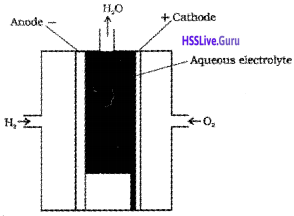• High efficiency
• Pollution free

Question 14.
Certain cells are given below.

1. In Daniel cell, if Zn electrode is replaced by Mg what happens? Why?
2. Zinc displaces copper from copper sulphate solution while copper doesn’t replace zinc from zinc sulphate solution. Justify your answer.

1. If Zinc electrode is replaced by Mg, a greater voltage (> 1.1 V) will be produced by the cell. Because Mg lies above Zn in electrochemical series.
Mg2+Mg) = -2.36 V
Zn2+Zn) = -0.76 V
i. e., Mg is a stronger reducing agent than Zn.

2. Zinc lying above hydrogen in the electrochemical series has relatively greater tendency to lose electrons. Hence Zn is capable of displacing hydrogen from acids. Moreover, a more active metal Zn, displaces, a less reactive copper from the salt solution. But Cu, being less active than Zn cannot displace Zn from its salt, ZnSO4.

### Plus Two Chemistry Electrochemistry NCERT Questions and Answers

Question 1.
Depict the galvanic cell in which the reaction Zn(s) + 2Ag+(aq) → Zn2+ + 2Ag(s) takes place. Further show:

1. Which of the electrodes is negatively charged?
2. The carriers of current in the cell.
3. Individual reaction at each electrode.

The electrochemical cell which will involve the given cell reaction is depicted as Zn(s) | Zn2+(aq) || Ag+(aq) | Ag(s)

1. In this cell, the electrode Zn(s) | Zn2+(aq) will act as negative terminal and Ag | Ag+(aq) electrode will act as positive terminal.

2. The conventional current will flow from silver to zinc electrode in the external circuit.

3. Individual reaction at each electrode:

• Cathodic reaction: 2Ag+(aq) + 2e → 2Ag(s)
• Anodic reaction: Zn(s) → Zn2+(aq) + 2eQuestion 2.
The resistance of a conductivity cell containing 0.001
M KCl solution at 298 K is 1500 Ω. What is the cell constant if conductivity of 0.001 M KCl solution at 298 K is 0.146 × 10-3 S cm-1?
Cell constant = $$\frac{\text { Conductivity }}{\text { Conductance }}$$
i.e., Cell constant = Conductivity × Resistance = 0.146 × 10-3 S cm-1 × 1500 Ω
= 0.219 cm-1

Question 3.
How much electricity in Faraday is required to produce

1. 20.0 g of Ca from molten CaCl2?
2. 40.0 g of Al from molten Al2O3?

1. The electrode reaction is
Ca2+(aq) + 2e → Ca(s)
Electricity required to produce 40 g Ca = 2 F
Electricity required to produce 20 g Ca = 1 F

2. The electrode reaction is
Al3+(aq) + 3e → Al(s)
Electricity required to produce 27 g of Al = 3 F
Electricity required to produce 40 g Al = $$\frac{3 F \times 40}{27}$$ = 4.44 F

Question 4.
The conductivity of 0.20 M solution of KCl at 298 K is 0.0248 S cm-1. Calculate the molar conductivity.